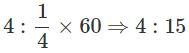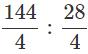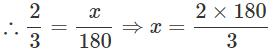Courses

# Test: Ratio And Proportion - 3

## 20 Questions MCQ Test Mathematics (Maths) Class 6 | Test: Ratio And Proportion - 3

Description
This mock test of Test: Ratio And Proportion - 3 for Class 6 helps you for every Class 6 entrance exam. This contains 20 Multiple Choice Questions for Class 6 Test: Ratio And Proportion - 3 (mcq) to study with solutions a complete question bank. The solved questions answers in this Test: Ratio And Proportion - 3 quiz give you a good mix of easy questions and tough questions. Class 6 students definitely take this Test: Ratio And Proportion - 3 exercise for a better result in the exam. You can find other Test: Ratio And Proportion - 3 extra questions, long questions & short questions for Class 6 on EduRev as well by searching above.
QUESTION: 1

### Identify the ratio of 4 seconds and 1/4 minute from the following.

Solution:

Convert 14 minute to seconds and find the ratio.QUESTION: 2

### A poster is 1.5 cm long and 7.5 cm wide. Which of the following is the ratio (in lowest terms) of the length and perimeter of the poster?

Solution:

Perimeter = 2(L+B)

P = 2(1.5+7.5) = 18

Length:Perimeter = 1.5:18

L:P = 1:12

QUESTION: 3

### The length and width of a tape are 2 m and 28 cm. What is their ratio?

Solution:
QUESTION: 4

What is the new ratio obtained by adding 4 to the antecedent and 2 to the consequent of the ratio 3:8?

Solution:
QUESTION: 5

In a cricket coaching camp, 1200 children are trained out of which 900 are selected for various matches. What is the ratio of non-selected children to the total number of children?

Solution:
QUESTION: 6

What is the condition for two ratios to be equal?

Solution:
QUESTION: 7

What are the extremes of the proportion 9:3::36:12?

Solution:

Extremes are the end terms. So, 9 and 12 area the extremes of the given proportion.

QUESTION: 8

What is the value of x in 12:3: :x:1?

Solution:

12:3::x:1  ⇒3x  =12 ⇒ x = 12/3 = 4

QUESTION: 9

Which of the following is a proportion?

Solution:

In a proportion, product of extremes is equal to product of means.

QUESTION: 10

What is the simplest form of the ratio 144:28?

Solution:(Since H.C.F. of 144 and 28=4)

QUESTION: 11

Find the mean proportion of 25:10::10:4.

Solution:

25:10::10:4 102=25 × 4 [ Since b2 = ac ]
∴ 10 is the mean proportion.

QUESTION: 12

If x : y : : y : z, identify the correct statement from among the following.

Solution:

x:y::y:z ⇒y2=xz

QUESTION: 13

Fill in the box so that the three numbers,32 and 64 are in proportion.

Solution:
QUESTION: 14

The ratios 6:3 and 5 :15 are given. Which of the following is true about them?

Solution:
QUESTION: 15

The first, second and the third terms of a proportion are 5,120 and 40. What is the fourth term?

Solution:
QUESTION: 16

The first, third and the fourth terms of a proportion are 6, 12 and 36. What is the second term?

Solution:

The ratio of boys and girls = 2:3 Girls =180 Let the number of boys be x=120 ⇒ Boys = 120

QUESTION: 17

The ratio of number of boys to number of girls in a tutorial is 2:3. If there are 180 girls, what is the number of boys?

Solution:

Given ratio =3:5 lemons = 12 (i.e.,3×4 )water = ? 3 × 4 : 5 × 4 ∴The required number of cups of water =20

QUESTION: 18

The ratio of lemons to number of cups of water to be mixed to prepare lemon juice is 3 :5. For 12 lemons what is the number of cups of water required?

Solution:

Number of players in a cricket team = 11 Wicketkeeper = 1
∴  Ratio =11:1

QUESTION: 19

In a cricket team what is the ratio of total number of players to the number of wicket keepers?

Solution:

Total number of players in a cricket team: 11
Number of  Wicket keeper : 1
Ratio 11:1

QUESTION: 20

In the word GEOMETRY, what is the ratio of number of consonants to the number of vowels?

Solution: